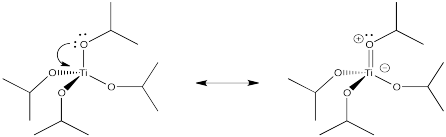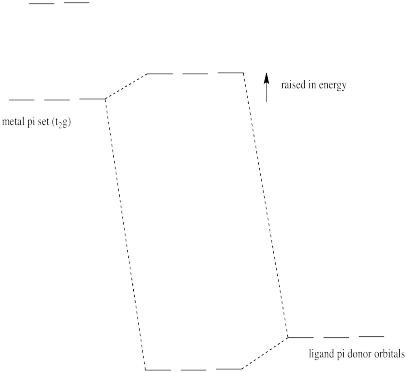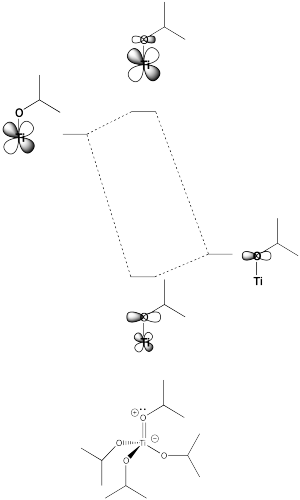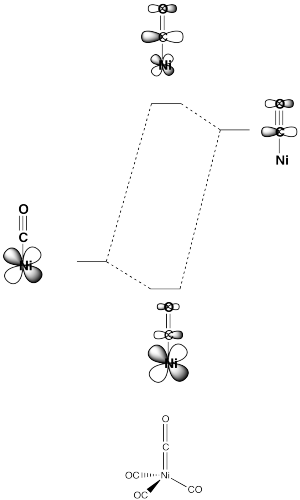# 6.1.1: The Spectrochemical Series

$$\newcommand{\vecs}{\overset { \rightharpoonup} {\mathbf{#1}} }$$ $$\newcommand{\vecd}{\overset{-\!-\!\rightharpoonup}{\vphantom{a}\smash {#1}}}$$$$\newcommand{\id}{\mathrm{id}}$$ $$\newcommand{\Span}{\mathrm{span}}$$ $$\newcommand{\kernel}{\mathrm{null}\,}$$ $$\newcommand{\range}{\mathrm{range}\,}$$ $$\newcommand{\RealPart}{\mathrm{Re}}$$ $$\newcommand{\ImaginaryPart}{\mathrm{Im}}$$ $$\newcommand{\Argument}{\mathrm{Arg}}$$ $$\newcommand{\norm}{\| #1 \|}$$ $$\newcommand{\inner}{\langle #1, #2 \rangle}$$ $$\newcommand{\Span}{\mathrm{span}}$$ $$\newcommand{\id}{\mathrm{id}}$$ $$\newcommand{\Span}{\mathrm{span}}$$ $$\newcommand{\kernel}{\mathrm{null}\,}$$ $$\newcommand{\range}{\mathrm{range}\,}$$ $$\newcommand{\RealPart}{\mathrm{Re}}$$ $$\newcommand{\ImaginaryPart}{\mathrm{Im}}$$ $$\newcommand{\Argument}{\mathrm{Arg}}$$ $$\newcommand{\norm}{\| #1 \|}$$ $$\newcommand{\inner}{\langle #1, #2 \rangle}$$ $$\newcommand{\Span}{\mathrm{span}}$$$$\newcommand{\AA}{\unicode[.8,0]{x212B}}$$

Another factor that plays a key role in whether a transition metal complex is high- or low-spin is the nature of the ligands. The d orbital energy splitting is influenced by how strongly the ligand interacts with the metal. Ligands that interact weakly only produce little change in the d orbital energy levels, whereas ligands that interact strongly produce a larger change in d orbital energy levels. The spectrochemical series is a list of ligands based on the strength of their interaction with metal ions. Ligands are often listed from weaker to stronger ligands, something like this:

I < Br < S2− < SCN < Cl < NO3 < N3 < F < OH < C2O42− < H2O < NCS < CH3CN < py < NH3

< en < bipy < phen < NO2 < PPh3 < CN < CO

in which py = pyridine; en = ethylenediamine; bipy = 2,2'-bipyridine; phen = 1,10-phenanthroline; SCN means the ligand is bound via sulfur and NCS via nitrogen. The list can vary from one metal ion to another, since some ligands bind preferentially to certain metals (as seen in hard and soft acid and base chemistry).

##### Exercise $$\PageIndex{1}$$

What empirical trends can you see within the spectrochemical series? Are there any factors that make something a stronger field ligand?

The weaker donors include halides and oxygen donors. Nitrogen atom donors are mostly a little stronger than that. The strongest donors include carbon donors, especially with pi bonds.

The d orbitals that rise in energy in the presence of a ligand can be thought of as forming an antibonding molecular orbital combination with an orbital on the ligand. In addition, there would also be a bonding combination for this interaction. That bonding orbital would be more like the initial ligand orbital. This premise is based on the idea that a ligand orbital is initially lower in energy than the metal orbital, so a bonding combination between these two orbitals is more like the initial ligand orbital, both in energy and location. The d orbital is initially higher in energy than the ligand orbital, so an antibonding combination between these two orbitals is more like the initial d orbital, both in energy and location.

• When orbitals on two different kinds of atoms combine, the antibonding orbital is considered to be more like the orbital that was initially at higher energy.
• In this case, it is still close to a d orbital in energy, location, and shape.
• When orbitals on two different kinds of atoms combine, the bonding orbital is considered to be more like the orbital that was initially at lower energy.
##### Exercise $$\PageIndex{2}$$

Suppose a ligand has more than one lone pair on the donor atom. The donor atom could share an extra pair of electrons with the metal, to form a double bond. This type of interaction is called pi-donation, because a $$\pi$$ bond is formed (not to be confused with sigma donation from a $$\pi$$ bond, as in alkene binding). Show an example using titanium isopropoxide $$Ti(OiPr)_4$$.##### Exercise $$\PageIndex{3}$$

In an octahedral environment, three of the d orbitals were not affected by sigma donation from the ligands. Show what happens to the energy level of these d orbitals in the presence of a $$\pi$$ donor.

Pi donation raises the $$t_{2g}$$ electrons (the d electrons of proper symmetry for pi overlap with the ligands in an octahedral geometry).##### Exercise $$\PageIndex{4}$$

Some ligands can accept a pair of electrons from the metal. An example is a carbonyl complex, which has a C=O $$\pi$$ antibonding orbital that can interact with a d orbital.

1. Show the antibonding orbital on the carbonyl (CO) ligand.
2. Show how a metal d orbital can interact with this orbital.
##### Exercise $$\PageIndex{5}$$

In the previous problem, a lower-energy atomic orbital on the metal interacts with a higher-energy antibonding orbital on the ligand. Show what happens to the energies of these two orbitals when they interact with each other.

Some of the trends we see in the spectrochemical series arise from pi-donating and pi-accepting effects in the ligand. Ligands that have additional lone pairs (other than the one that sigma donates) are $$\pi$$ donors. $$\pi$$ donors raise the otherwise non-bonding $$t_{2g}$$ orbitals, because the lone pair on the ligand forms a $$\pi$$ bond with the metal. The $$t_{2g}$$ orbitals and the ligand lone pair orbitals form two new orbitals. The antibonding orbital is closer in energy to the high-energy d orbitals. The bonding orbital is closer in energy to the low-energy ligand orbital.

• $$\pi$$ donation raises the $$t_{2g}$$ set of d orbitals in energy.
• As a result, the d orbital splitting gets smaller.
• Also as a result, a complex with $$\pi$$ donation is a little less stable than a complex without $$\pi$$ donation.

This type of interaction can be seen in the following pictures (a tetrahedral case).On the other hand, ligands in which the donor atom is already $$\pi$$ bonding to another atom can accept $$\pi$$ donation from the metal. This happens by donating an electron pair from a metal $$t_{2g}$$ orbital into a $$\pi*$$ orbital on the ligand. In this case, because the $$\pi*$$ is an antibonding orbital, it is higher in energy than the metal d orbital (or the $$t_{2g}$$ orbital). The resulting bonding orbital is more like the lower energy metal orbital, whereas the resulting antibonding orbital is more like the higher energy $$\pi*$$ orbital on the ligand.

• $$\pi$$ accepting ligands lower the $$t_{2g}$$ set of d orbitals in energy.
• As a result, the d orbital splitting gets larger.
• Also as a result, a complex with a $$\pi$$ accepting ligand is a little more stable than a complex without a $$\pi$$ accepting ligand.

This type of interaction can be seen in the following case (a tetrahedral complex).The spectrochemical series gets its name because of a shift in a band of the UV-Vis spectrum when two similar complexes are compared that have two different ligands. The effect of the ligand on the d orbital splitting has an effect on the wavelength of light associated with a d orbital (filled) to d orbital (empty) electronic transition. This transition is actually not associated with a major absorption. However, because it often occurs in the region of visible light, it is associated with colored transition metal complexes.

##### Exercise $$\PageIndex{6}$$

Explain what happens to the wavelength of light absorbed for the d-d transition when a chloride ligand on a metal complex is replaced with a hydroxide ligand.